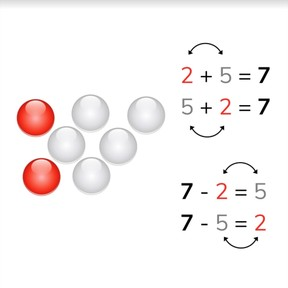Addition and subtraction to 10 using fact families

# Addition and subtraction to 10 using fact families

No account needed.8,000 schools use Gynzy92,000 teachers use Gynzy1,600,000 students use Gynzy

## General

Students learn to add and subtract numbers to 10 using fact families. They learn that each addition problem has a matching inverse subtraction problem.

1.OA.B.3
1.OA.B.4

## Introduction

Start by decomposing the numbers given into their respective amounts of beads. Then decompose the numbers showing their number bonds. Students can decompose the number with you with blocks at their tables. You can also ask students to decompose a few numbers out loud in which you ask them to decompose a given number. Example: I decompose the number 5 into 4 and .... Then take a box and a few erasers (or other object). Tell students that you have 6 in your hand and are placing 5 into the box. Ask students which math problem represents that (6 - 5). Repeat this with different quantities. Also do this with addition problems, for example 2 erasers in your hand, and you add 3 from the box. (2 + 3). Then determine which math problem is shown on the board. You see 4 dogs. 3 are outside of the dog field and 1 is inside. Discuss why the other problems are incorrect (for example 3 - 1 is wrong, because the total amount of dogs is 4, not 3).

## Instruction

Show students the image of 7 beads, of which 2 are red and 5 are white. Tell students that you can make addition and subtraction problems that belong together. The numbers stay the same, but their position in the math problem gets swapped. In this case, 2 + 5 = 7, 5 + 2 = 7, 7 - 2 = 5, and 7 - 5 = 2 belong together. Practice this with other numbers. Use the number bonds to show how 5 can be decomposed. Using these numbers you can make four math problems, two addition and two subtraction problems. Tell students that they can think about the beads, 1 white and 4 red. Practice this with other numbers. Then challenge students to try to do this without beads or number bonds visible on the board.

Check that students are able to use fact families with numbers to 10 for addition and subtraction problems by asking the following questions:
- 3 + 4 = 7, Which other addition problem is the partner to this one?
- 9 - 3 = 6, so we then know that 9 - 6 = ...?
- 5 + 3 = 8, so we then know that 8 - 3 = ...?
- Can you swap the numbers in an addition problem?
- Which numbers can you swap in a subtraction problem?
- If you want to make a subtraction problem out of an addition problem, which number do you start with?

## Quiz

Students are first given problems in which they can count the objects as support. They are then given number bonds to use in solving the addition and subtraction problems.

## Conclusion

Discuss the learning goal with students, and emphasize that by being able to use the inverse and substitution strategies, that they make math problems easier and faster to solve. To close the lesson, solve two problems that belong to the given image as a class. Then repeat, but with a problem in which you can't see the number division.

## Teaching tips

Students who have difficulty with this learning goal can make use of MAB blocks, or manipulatives or a rekenrek. Ask them to decompose the number 7 into 3 and 4 blocks. Have them set the blocks down and tell which addition problems they can make with these blocks (3 + 4 = 7and 4 + 3 = 7). Then ask what is left if you take 3 blocks away from 7 (7 - 3 =?) and what is left if they take 4 blocks away from 7 (7 - 4 =?). Remind them that they don't need to recalculate because they already know how many blocks there are as a result of the addition problem.

## Instruction materials

Box and some erasers (or other object)
Optional: MAB blocks, manipulatives, rekenrek

### The online teaching platform for interactive whiteboards and displays in schools

• Save time building lessons

• Manage the classroom more efficiently

• Increase student engagement